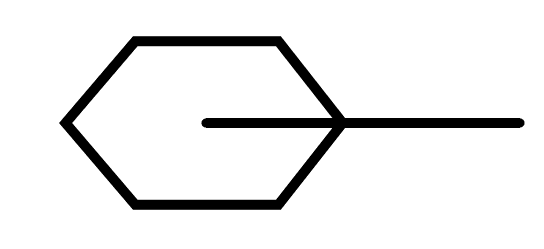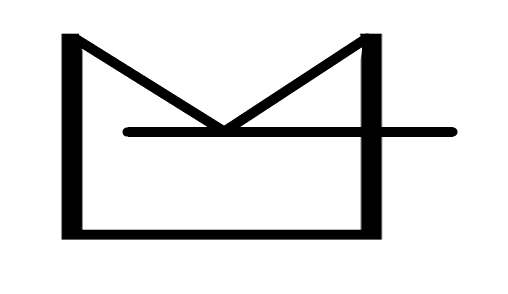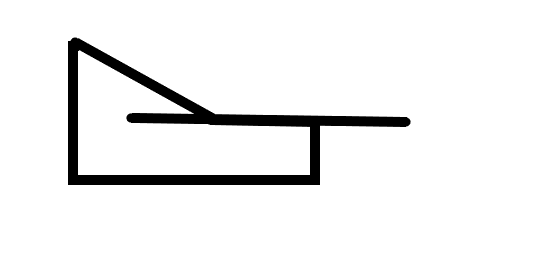多边形_多边形建模 - CSDN

• 方法一：扫描法(使用于任意多边形) 通常情况下，当射线与多边形的交点个数是奇数时，Q在多边形内，是偶数时，Q在多边形外。 通常将射线设为水平向右，那么就有一些特殊情况值得考虑 1.射线与多边形的顶点相交，这...
方法一：扫描法(使用于任意多边形)

通常情况下，当射线与多边形的交点个数是奇数时，Q在多边形内，是偶数时，Q在多边形外。

通常将射线设为水平向右，那么就有一些特殊情况值得考虑

1.射线与多边形的顶点相交，这是交点只能计算一个。2.射线与多边形顶点的交点不应该被计算3.射线与多边形的一条边重合，这条边应该被忽略算法描述：首先，对于多边形的水平边不做考虑，其次，对于多边形的顶点和射线相交的情况，如果该顶点时其所属的边上纵坐标较大的顶点，则计数，否则忽略该点，最后，对于Q在多边形上的情形，直接判断Q是否属于多边形。

时间复杂度分析：O(n)

注意：点的输入必须有顺序，不是顺时针就是逆时针

附上代码：


#include<iostream>
#include<cstdio>

using namespace std;

const int maxn=110;
const double eps=1e-5;

struct point{
double x,y;
};
point poly[maxn];

int n,q;

bool onsegment(point pi,point pj,point Q)
{
if((Q.x-pi.x)*(pj.y-pi.y)==(pj.x-pi.x)*(Q.y-pi.y)&&min(pi.x,pj.x)<=Q.x&&Q.x<=max(pi.x,pj.x)&&min(pi.y,pj.y)<=Q.y&&Q.y<=max(pi.y,pj.y)){
return true;
}else{
return false;
}
}

bool insidepolygon(point p)
{
int counter=0;
double xinters;
point p1,p2;
p1=poly;
for(int i=1;i<=n;i++){
p2=poly[i%n];
if(onsegment(p1,p2,p)){
return true;
}
if(p.y>min(p1.y,p2.y)){
if(p.y<=max(p1.y,p2.y)){
if(p.x<=max(p1.x,p2.x)){
if(p1.y!=p2.y){
xinters=(p.y-p1.y)*(p2.x-p1.x)/(p2.y-p1.y)+p1.x;
if(p1.x==p2.x||p.x<=xinters){
counter++;
}
}
}
}
}
p1=p2;
}
if(counter%2==0){
return false;
}
return true;
}

int main()
{
point p;
scanf("%d%d",&n,&q);
for(int i=0;i<n;i++){
scanf("%lf%lf",&poly[i].x,&poly[i].y);
}
for(int i=0;i<q;i++){
scanf("%lf%lf",&p.x,&p.y);
if(insidepolygon(p)){
printf("within\n");
}else{
printf("outside\n");
}
}
return 0;
}

方法二：叉乘判别法(只适用于凸多边形)

设这个多边形的边数为n。选一条边作为1号边，然后按照顺时针或者逆时针给每条边编号，连接第i(i=1,2,3......,n)条边的第一个端点和要测试的点得到一个向量vi。连接第一个端点与第二个端点得到向量ui，都以第一个端点为起点，作叉积vi*ui，记录结果。除了第一条边以外，都与前一次运算得到的叉积作乘积。如果为正则继续判断，知道遍历所有边，若全部满足，则证明点在多边形内，否则，证明点在多边形外。

#include<iostream>
#include<cstdio>

using namespace std;

const int maxn=110;

struct point{
double x,y;
};
point poly[maxn];
int n,q;

double multi(point p1,point p2,point p0)
{
return (p1.x-p0.x)*(p2.y-p0.y)-(p2.x-p0.x)*(p1.y-p0.y);
}

bool isinside(point p)
{
double pre,now;
for(int i=0;i<n;i++){
now=multi(p,poly[i],poly[(i+1)%n]);
if(i>0){
if(pre*now<0){
return 0;
}
}
pre=now;
}
return true;
}

int main()
{
scanf("%d%d",&n,&q);
for(int i=0;i<n;i++){
scanf("%lf%lf",&poly[i].x,&poly[i].y);
}
point p;
for(int i=0;i<q;i++){
scanf("%lf%lf",&p.x,&p.y);
if(isinside(p)){
printf("Yes\n");
}else{
printf("No\n");
}
}
return 0;
}

方法三：角度和的判断法(适用于任意多边形)

对于平面多边形来说，连接多边形内点于多边形所有顶点所形成的所有角的角度在要求精度范围内应该等于360度，如果小于360度或者大于360度，则证明不在多边形中。

#include<iostream>
#include<cstdio>
#include<cmath>

using namespace std;

const int maxn=110;
const double pi=3.1415926;

struct point{
double x,y;
};
point poly[maxn];

int n,q;

double ang(double x1,double y1,double x2,double y2)
{
double ans=x1*x2+y1*y2;
double base=sqrt(x1*x1+y1*y1)*sqrt(x2*x2+y2*y2);
ans/=base;
return acos(ans);
}

int inpolygon(point p)
{
double angle=0;
for(int i=0;i<n;i++){
double x1=poly[i].x-p.x;
double y1=poly[i].y-p.y;
double x2=poly[(i+1)%n].x-p.x;
double y2=poly[(i+1)%n].y-p.y;
angle+=ang(x1,y1,x2,y2);
}
if(fabs(angle-2*pi)<0.000001){
return true;
}else{
return false;
}
}

int main()
{
scanf("%d%d",&n,&q);
for(int i=0;i<n;i++){
scanf("%lf%lf",&poly[i].x,&poly[i].y);
}
point p;
for(int i=0;i<q;i++){
scanf("%lf%lf",&p.x,&p.y);
if(inpolygon(p)){
printf("Yes\n");
}else{
printf("No\n");
}
}
return 0;
}


展开全文• 计算p1p2,p2p3的叉乘，阶乘大于0，则表示p3点在线段p1和p2的左侧，然后依次计算下一个前后所组成向量的阶乘，如果在计算时，出现负值，则此多边形是凹多边形，如果所有顶点计算完毕，其结果都大于0，则多边形是凸...
判定凸多边形：顶点凹凸性法
连续三个顶点p1,p2,p3。计算p1p2,p2p3的叉乘，阶乘大于0，则表示p3点在线段p1和p2的左侧，然后依次计算下一个前后所组成向量的阶乘，如果在计算时，出现负值，则此多边形是凹多边形，如果所有顶点计算完毕，其结果都大于0，则多边形是凸多边形。
判断点在凸多边形内外：
①：与判定凸多边形差不多，用判断点与多边形两顶点叉乘，都大于0，点在多边形内，小于0，点在多边形外。
②：水平/垂直交叉点数判别法（适用于任意多边形包括凹凸边形）
注意到如果从P作水平向左的射线的话，如果P在多边形内部，那么这条射线与多边形的交点必为奇数，如果P在多边形外部，则交点个数必为偶数（0也在内）。所以，我们可以顺序考虑多边形的每条边，求出交点的总个数。还有一些特殊情况要考虑。
判断线段在任意多边形内：
（1）首先，要判断一条线段是否在多边形内，先要判断线段的两个端点是否在多边形内。如果两个端点不全在多边形内，那么，线段肯定是不在多边形内的。
（2）其次，如果线段和多边形的某条边内交（两线段内交是指两线段相交且交点不在两线段的端点），则线段肯定不在多边形内。
（3）如果多边形的某个顶点和线段相交，则必须判断两相交交点之间的线段是否包含于多边形内。
求多边形重心：
以第一个顶点为基准，分别连接p[i]，p[i+1]，1<i<n。将多边形划分为若干个三角形，求出了每个三角形的重心，用叉积求三角形面积，对凸多边形和凹多边形都适用（因为值有正负）；作为二维的多边形，把面积作为权值，分别乘以重心坐标的X和Y值；分别将求出的X, Y值的加权平均数除以总面积，即多边形面积的重心坐标。
具体代码实现：
#include <iostream>
#include <stdlib.h>
#include <math.h>
#define MAXN 1000
#define offset 10000
#define eps 1e-8
#define zero(x) (((x)>0?(x):-(x))<eps)
#define _sign(x) ((x)>eps?1:((x)<-eps?2:0))

using namespace std ;

struct point{double x,y;}p[MAXN];
struct line{point a,b;};

double xmult(point p1,point p2,point p0)  //计算向量p1p2，p2p3的叉积
{
return (p1.x-p0.x)*(p2.y-p0.y)-(p2.x-p0.x)*(p1.y-p0.y);
}

int is_convex(int n,point* p)  //判定凸多边形,顶点按顺时针或逆时针给出,允许相邻边共线
{
int i,s={1,1,1};
for (i=0;i<n&&s|s;i++)
s[_sign(xmult(p[(i+1)%n],p[(i+2)%n],p[i]))]=0;
return s|s;
}
int is_convex_v2(int n,point* p)  //判定凸多边形,顶点按顺时针或逆时针给出,不允许相邻边共线
{
int i,s={1,1,1};
for (i=0;i<n&&s&&s|s;i++)
s[_sign(xmult(p[(i+1)%n],p[(i+2)%n],p[i]))]=0;
return s&&s|s;
}

int inside_convex(point q,int n,point* p)  //判点在凸多边形内或多边形边上,顶点按顺时针或逆时针给出
{
int i,s={1,1,1};
for (i=0;i<n&&s|s;i++)
s[_sign(xmult(p[(i+1)%n],q,p[i]))]=0;
return s|s;
}
int inside_convex_v2(point q,int n,point* p)  //判点在凸多边形内,顶点按顺时针或逆时针给出,在多边形边上返回0
{
int i,s={1,1,1};
for (i=0;i<n&&s&&s|s;i++)
s[_sign(xmult(p[(i+1)%n],q,p[i]))]=0;
return s&&s|s;
}

int inside_polygon(point q,int n,point* p,int on_edge=2)  //判点在任意多边形内,顶点按顺时针或逆时针给出,on_edge表示点在多边形边上时的返回值,offset为多边形坐标上限
{
point q2;
int i=0,count;
while (i<n)
for (count=i=0,q2.x=rand()+offset,q2.y=rand()+offset;i<n;i++)//随机取一个足够远的点q,以p为起点q为终点做射线L,依次对多边形的每条边进行考察
if( zero(xmult(q,p[i],p[(i+1)%n])) && (p[i].x-q.x)*(p[(i+1)%n].x-q.x)<eps && (p[i].y-q.y)*(p[(i+1)%n].y-q.y)<eps )
return on_edge;//点p在边上,返回on_edge
else if (zero(xmult(q,q2,p[i])))
break;//点q在射线pq2上，停止本循环，另取q2
else if
(xmult(q,p[i],q2)*xmult(q,p[(i+1)%n],q2)<-eps&&xmult(p[i],q,p[(i+1)%n])*xmult(p[i],q2,p[(i+1)%n])<-eps)
count++;
return count&1;
}
inline int opposite_side(point p1,point p2,point l1,point l2)
{
return xmult(l1,p1,l2)*xmult(l1,p2,l2)<-eps;
}
inline int dot_online_in(point p,point l1,point l2)
{
return zero(xmult(p,l1,l2))&&(l1.x-p.x)*(l2.x-p.x)<eps&&(l1.y-p.y)*(l2.y-p.y)<eps;
}
int inside_polygon(point l1,point l2,int n,point* p)  //判线段在任意多边形内,顶点按顺时针或逆时针给出,与边界相交返回1
{
point t[MAXN],tt;
int i,j,k=0;
if (!inside_polygon(l1,n,p)||!inside_polygon(l2,n,p))
return 0;
for (i=0;i<n;i++)
if (opposite_side(l1,l2,p[i],p[(i+1)%n])&&opposite_side(p[i],p[(i+1)%n],l1,l2))
return 0;
else if (dot_online_in(l1,p[i],p[(i+1)%n]))
t[k++]=l1;
else if (dot_online_in(l2,p[i],p[(i+1)%n]))//线段的某个端点在S上
t[k++]=l2;
else if (dot_online_in(p[i],l1,l2))
t[k++]=p[i];
for (i=0;i<k;i++)
for (j=i+1;j<k;j++)
{
tt.x=(t[i].x+t[j].x)/2;
tt.y=(t[i].y+t[j].y)/2;
if (!inside_polygon(tt,n,p))
return 0;
}
return 1;
}

point intersection(line u,line v)  //求多边形重心
{
point ret=u.a;
double t=((u.a.x-v.a.x)*(v.a.y-v.b.y)-(u.a.y-v.a.y)*(v.a.x-v.b.x))/((u.a.x-u.b.x)*(v.a.y-v.b.y)-(u.a.y-u.b.y)*(v.a.x-v.b.x));
ret.x+=(u.b.x-u.a.x)*t;
ret.y+=(u.b.y-u.a.y)*t;
return ret;
}
point barycenter(point a,point b,point c)
{
line u,v;
u.a.x=(a.x+b.x)/2;
u.a.y=(a.y+b.y)/2;
u.b=c;
v.a.x=(a.x+c.x)/2;
v.a.y=(a.y+c.y)/2;
v.b=b;
return intersection(u,v);
}
point barycenter(int n,point* p)
{
point ret,t;
double t1=0,t2;
int i;
ret.x=ret.y=0;
for (i=1;i<n-1;i++)
if (fabs(t2=xmult(p,p[i],p[i+1]))>eps)
{
t=barycenter(p,p[i],p[i+1]);
ret.x+=t.x*t2;
ret.y+=t.y*t2;
t1+=t2;
}
if (fabs(t1)>eps)
ret.x/=t1,ret.y/=t1;
return ret;
}

int main()
{
}


展开全文C++ 算法
• 给定多边形的顶点坐标（有序），让你来求这个多边形的面积，你会怎么做？ 我们知道，任意多边形都可以分割为N个三角形，所以，如果以这为突破点，那么我们第一步就是把给定的多边形，分割为数个三角形，分别求面积，...
给定多边形的顶点坐标（有序），让你来求这个多边形的面积，你会怎么做？
我们知道，任意多边形都可以分割为N个三角形，所以，如果以这为突破点，那么我们第一步就是把给定的多边形，分割为数个三角形，分别求面积，最后累加就可以了，把多边形分割为三角形的方式多种多样，在这里，我们按照如下图的方法分割：图1

（点如果是顺时针给出，有向面积为负，逆时针给出，有向面积为正）

对于图1而言，多边形的面积就是：
S(1->6)=S(1,2,3)+S(1,3,4)+S(1,4,5)+S(1,5,6)（对凸多边形同样适用）

如果我们不以多边形的某一点为顶点来划分三角形而是以任意一点，如下图，这个方法也是成立的：S = S_OAB + S_OBC + S_OCD + S_ODE + S_OEA。计算的时候，当我们取O点为原点时，可以简化计算。当O点为原点时，根据向量的叉积计算公式，各个三角形的面积计算如下：

设a(x1,y1),b(x2,y2)
以下均为向量：
oa(x1,y1),ob(x2,y2)
oa X ob由线性代数叉积知识得：
=x1y2-x2y1

S_OAB = 0.5*(A_x*B_y - A_y*B_x)   【(A_x，A_y)为A点的坐标】

S_OBC = 0.5*(B_x*C_y - B_y*C_x)

S_OCD = 0.5*(C_x*D_y - C_y*D_x)

S_ODE = 0.5*(D_x*E_y - D_y*E_x)

S_OEA = 0.5*(E_x*A_y - E_y*A_x)

点如果是顺时针给出，有向面积为负，逆时针给出，有向面积为正,OAB即为O>A>B,即OA向量XOB向量

无需担心点点坐标为正还是负，正负只与点给出的顺序有关，最终结果取绝对值即可。

题目：hdu2036     http://acm.hdu.edu.cn/showproblem.php?pid=2036

//输入必须是将点按顺序输入，顺时还是逆时程序会处理的
#include<cstdio>
using namespace std;
double ans;
int n;
struct Point{
int x,y;
}a;
int ret=0,f=1;char ch=getchar();
while(ch<'0'||ch>'9'){if(ch=='-')f=-f;ch=getchar();}
while(ch>='0'&&ch<='9') ret=ret*10+ch-'0',ch=getchar();
return ret*f;
}
int main(){
for(int i=1;i<=n;i++)
for(int i=2;i<=n;i++)            //取原点为辅助点
ans+=(double)(a[i].x*a[i-1].y-a[i].y*a[i-1].x)/*记得求平行四边形面积公式吗*//2.0;
//求三角形面积。全加起来就好了，因为...面积有方向（正负性），自己会消掉的
ans+=(double)(a.x*a[n].y-a.y*a[n].x)/2.0;//第1和第n个点单独处理
if(ans<0.0) ans=-ans;//顺时针与逆时针输入结果互为相反数
printf("%lf",ans);
return 0;
}

//起点为（0.0）时
#include <iostream>
#include <stdio.h>
#include <algorithm>
#include <cmath>
#define fre freopen("C:\\Users\\Dell\\Desktop\\in.txt", "r", stdin);
using namespace std;
struct area{
double x;double y;
};
int main(){
//fre;
int t,n;
double sum;
struct area a;
cin>>t;
while(t--){
sum=0.0;
cin>>n;
for(int i=0;i<n;i++)
cin>>a[i].x>>a[i].y;
for(int i=1;i<n-1;i++)
sum+=(a[i].x*a[i+1].y-a[i+1].x*a[i].y);
sum=fabs(sum/2.0);     //求double绝对值用fabs,float:fabsf,int:abs
printf("%.6f\n",sum);

}
return 0;
}



展开全文• ## 多边形切割

千次阅读 2018-06-20 14:30:50
之前基本上遇到的多边形切割问题都是凸多边形问题，而针对凹多边形的切割问题却很少。偶然发现一个做得特别棒的滑动切割的游戏，游戏中主要是使用多边形切割以及多边形碰撞算法。针对多边形切割的实现做了一下研究，...
      之前基本上遇到的多边形切割问题都是凸多边形问题，而针对凹多边形的切割问题却很少。偶然发现一个做得特别棒的滑动切割的游戏，游戏中主要是使用多边形切割以及多边形碰撞算法。针对多边形切割的实现做了一下研究，现在把实现跟大家分享一下。      给定任意一个多边形以及一条线段，如果多边形被线段切割，计算切割后产生的多个多边形。实现的算法思想如下：      1、求多边形每一条边跟线段的交点，将交点插入多边形的顶点序列，得到新的顶点序列。      2、如果交点超过一个，多边形就有可能被切割。      3、先判断从第一个交点开始，线段是处于多边形内部还是多边形外部，找出距离交点最近的另一个交点，如果另一个交点是最后一个交点并且这两个交点连线的中点在多边形外，那么当前交点之后线段开始处于多边形内如果另一个交点是最后一个交点并且这两个交点连线的中点在多边内部，那么当前交点之后线段开始处于多边形外部如果另一个交点当前交点的下一个交点并且这两个交点连线的中点在多边内部，那么当前交点之后线段开始处于多边形内部如果另一个交点当前交点的下一个交点并且这两个交点连线的中点在多边外部，那么当前交点之后线段开始处于多边形外部      4、从第一个交点开始对新的顶点序列中的点开始拆分，如果多边形被切割了，那么当前交点一定是分割之后两个多边形的交点，在遇到下一个交点之前，原来多边形的顶点需要根据当前线段的状态来处理，如果当前线段处于多边形内部，这些点会被分割给新的多边形，如果线段在多边形外部，那么这些点还属于原多边形。      5、遇到新的交点，根据线段的状态来判断，如果线段之前是处于多边形内部，那么当前交点个前一个交点的连线将原多边形分割得到了新的多边形，并且接下来线段的状态会发生变化，线段开始处于多边形外部。如果线段之前处于多边形外部，那么从当前交点开始，线段开始处于多边形内部，当前交点和下一个交点将会分割元多边形，产生新的多边形。算法使用typescript实现如下：//求两条线段的交点
//返回值：[n,p] n:0相交，1在共有点，-1不相交  p:交点
public static lineCrossPoint(p1:cc.Vec2,p2:cc.Vec2,q1:cc.Vec2,q2:cc.Vec2){
let a = p1,b = p2,c = q1,d = q2;
let s1,s2,s3,s4;
let d1,d2,d3,d4;
let p:cc.Vec2 = new cc.Vec2(0,0);
d1=this.dblcmp(s1=this.ab_cross_ac(a,b,c),0);
d2=this.dblcmp(s2=this.ab_cross_ac(a,b,d),0);
d3=this.dblcmp(s3=this.ab_cross_ac(c,d,a),0);
d4=this.dblcmp(s4=this.ab_cross_ac(c,d,b),0);

//如果规范相交则求交点
if ((d1^d2)==-2 && (d3^d4)==-2)
{
p.x=(c.x*s2-d.x*s1)/(s2-s1);
p.y=(c.y*s2-d.y*s1)/(s2-s1);
return [0,p];
}

//如果不规范相交
if (d1==0 && this.point_on_line(c,a,b)<=0)
{
p=c;
return [1,p];
}
if (d2==0 && this.point_on_line(d,a,b)<=0)
{
p=d;
return [1,p];
}
if (d3==0 && this.point_on_line(a,c,d)<=0)
{
p=a;
return [1,p];
}
if (d4==0 && this.point_on_line(b,c,d)<=0)
{
p=b;
return [1,p];
}
//如果不相交
return [-1,null];
}

//两条线段是否跨立
//即非平行
public static isLineSegmentCross(P1:cc.Vec2,P2:cc.Vec2,Q1:cc.Vec2,Q2:cc.Vec2)
{
if(
((Q1.x-P1.x)*(Q1.y-Q2.y)-(Q1.y-P1.y)*( Q1.x-Q2.x)) * ((Q1.x-P2.x)*(Q1.y-Q2.y)-(Q1.y-P2.y)*(Q1.x-Q2.x)) < 0 ||
((P1.x-Q1.x)*(P1.y-P2.y)-(P1.y-Q1.y)*(P1.x-P2.x)) * ((P1.x-Q2.x)*(P1.y-P2.y)-(P1.y-Q2.y)*( P1.x-P2.x)) < 0
)
{
return true;
}
return false;
}

//求a点是不是在线段上，>0不在，=0与端点重合，<0在。
public static point_on_line(a,p1,p2)
{
return this.dblcmp(this.dot(p1.x-a.x,p1.y-a.y,p2.x-a.x,p2.y-a.y),0);
}
//点发出的右射线和线段的关系
// 返回值: -1:不相交, 0:相交, 1:点在线段上
public static rayPointToLine(point:cc.Vec2,linePA:cc.Vec2,linePB:cc.Vec2){
// 定义最小和最大的X Y轴值
let minX = Math.min(linePA.x,linePB.x);
let maxX = Math.max(linePA.x,linePB.x);
let minY = Math.min(linePA.y,linePB.y);
let maxY = Math.max(linePA.y,linePB.y);

// 射线与边无交点的其他情况
if (point.y < minY || point.y > maxY || point.x > maxX) {
return -1;
}

// 剩下的情况, 计算射线与边所在的直线的交点的横坐标
let x0 = linePA.x + ((linePB.x - linePA.x) / (linePB.y - linePA.y)) * (point.y - linePA.y);
if(x0 > point.x)
{
return 0;
}
if(x0 == point.x)
{
return 1;
}
return -1;
}

//点和多边形的关系
//返回值: -1:在多边形外部, 0:在多边形内部, 1:在多边形边线内, 2:跟多边形某个顶点重合
public static relationPointToPolygon(point:cc.Vec2,polygon:cc.Vec2[])
{
let count = 0;
for(let i = 0;i<polygon.length;++i)
{
if(polygon[i].equals(point))
{
return 2;
}

let pa = polygon[i];
let pb = polygon;
if(i < polygon.length -1)
{
pb = polygon[i+1];
}

let re = this.rayPointToLine(point,pa,pb);
if(re == 1)
{
return 1;
}
if(re == 0)
{
count++;
}
}
if(count %2 == 0)
{
return -1;
}
return 0;
}

//线段对多边形进行切割
//返回多边形数组
//如果没有被切割，返回空数组
public static lineCutPolygon(pa:cc.Vec2,pb:cc.Vec2,polygon:cc.Vec2[]){
let ret:Array<cc.Vec2[]> = [];

let points:cc.Vec2[] = [];
let pointIndex:number[] = [];

//将所有的点以及交点组成一个点序列
for(let i = 0;i<polygon.length;++i)
{
points.push(polygon[i]);

let a = polygon[i];
let b = polygon;
if(i < polygon.length -1) b = polygon[i+1];

let c = this.lineCrossPoint(pa,pb,a,b);
if(c == 0){
pointIndex.push(points.length);
points.push(c as cc.Vec2);
}
else if(c > 0)
{
if((c as cc.Vec2).equals(a))
{
pointIndex.push(points.length-1);
}
else
{
pointIndex.push(points.length);
}
}
}
if(pointIndex.length > 1)
{
//准备从第一个交点开始拆，先弄清楚第一个交点是由外穿内，还是内穿外
let cp0 = points[pointIndex];
let cp1 = points[pointIndex];

let r = this.relationPointToPolygon(new cc.Vec2((cp0.x + cp1.x)/2,(cp0.y+cp1.y)/2),polygon);
let inPolygon:boolean = r >=0;
if(pointIndex.length > 2 && cc.pDistance(cp0,cp1) > cc.pDistance(cp0,points[pointIndex[pointIndex.length-1]]))
{
cp1 = points[pointIndex[pointIndex.length-1]];
r = this.relationPointToPolygon(new cc.Vec2((cp0.x + cp1.x)/2,(cp0.y+cp1.y)/2),polygon);
inPolygon = r <0;
}

let firstInPolygon = inPolygon;//起始点是从外面穿到里面

let index = 0;
let startIndex = pointIndex[index];
let oldPoints = [];
let newPoints = [];
let count = 0;

oldPoints.push(points[startIndex]);
if(inPolygon)
{
newPoints.push(points[startIndex]);
}

index++;
count++;
startIndex++;

while(count < points.length)
{
if(startIndex == points.length) startIndex = 0;
let p = points[startIndex];
if(index >= 0 && startIndex == pointIndex[index])
{
//又是一个交点
index++;
if(index >= pointIndex.length) index = 0;
if(inPolygon){
//原来是在多边形内部
//产生了新的多边形
newPoints.push(p);
ret.push(newPoints);
newPoints = [];
}
else
{
//开始新的多边形
newPoints = [];
newPoints.push(p);
}
oldPoints.push(p);
inPolygon = !inPolygon;
}
else
{
//普通的点
if(inPolygon)
{
newPoints.push(p);
}
else
{
oldPoints.push(p);
}
}
startIndex++;
count++;
}
if(inPolygon)
{
if(!firstInPolygon && newPoints.length > 1)
{
//如果起始点是从里面穿出去，到这里跟起始点形成闭包
newPoints.push(points[pointIndex]);
ret.push(newPoints);
}
else
{
//结束了，但是现在的状态是穿在多边形内部
//把newPoints里面的回复到主多边形中
for(let i = 0;i<newPoints.length;++i)
{
oldPoints.push(newPoints[i]);
}
}

}

ret.push(oldPoints);
}
return ret;
}

private static ab_cross_ac(a,b,c) //ab与ac的叉积
{
return this.cross(b.x-a.x,b.y-a.y,c.x-a.x,c.y-a.y);
}
private static dot(x1,y1,x2,y2){
return x1*x2+y1*y2;
}
private static cross(x1,y1,x2,y2){
return x1*y2 - x2*y1;
}
private static dblcmp(a:number,b:number)
{
if (Math.abs(a-b)<=0.000001) return 0;
if (a>b) return 1;
else return -1;
}
展开全文• 多边形游戏”是一款单人益智游戏。 游戏开始时，给定玩家一个具有N个顶点N条边（编号1-N）的多边形，如图1所示，其中N = 4。 每个顶点上写有一个整数，每个边上标有一个运算符+（加号）或运算符*（乘号）。 第一步...
• ## 多边形扩展算法

千次阅读 2017-06-20 17:00:11扩展 算法 多边形
• 今天是5月14日，我们按照规定，给出几道答案等于14的题目。 （一） 一个正七边形有多少条对角线？（7个点之间两两连线，可以连接出7*6/2=21条线段，其中有七条是正七边形的边，那么，剩余线段的数量就是正七边形...
• ## 多边形的质心计算

千次阅读 2020-05-20 15:12:45
多边形实体的质心计算 难度指数：★★☆☆☆ 前情回顾 OK，第7篇运用了多边形三角剖分+向量叉积的方式计算多边形面积。初步见到了三角形剖分化繁为简的能力，这一篇算是此种算法思想的延伸——质心计算。 质心坐标...空间计算 向量
• 给定一个n多边形，要求用n-3条不相交的对角线把它分成n-2个三角形。求有多少种不同的方法。 分析 为什么是n-3条不相交的对角线？ n多边形有n个顶点，依次将其编号为V1、V2、V3、…、Vn。 从V1号到V3号连线，分成...
• ## 多边形重心的计算

千次阅读 2019-07-13 20:08:47
设平面上有n个点（xi，yi）(i=1、2、3……n)，其多边形重心G（x，y）为: 这是求多边形最简单直观的方法。可以直接利用数据点的x, y坐标就能求图形重心。但是缺陷在于没有对离散数据点所围图形做任何处理和分析,精度...
• ## 任意多边形的面积公式

万次阅读 多人点赞 2012-07-31 16:22:12
设Ω是m边形(如下图)，顶点沿边界正向排列，，坐标依次为...多边形计算公式的计算和原点的选取没有关系，通常可以选点（0,0）或者多边形的第一个点（这个时候比较直观了，看起来就是把多边形分成一个个三角形和加起来，
• 也可以访问多边形内最短路径页（shortest-path-through-polygonpage）！ 图 1 图1显示了一个具有14条边的凹多边形。我们要判断红色点是否在多边形内。 解决方案是将测试点的Y坐标与多边形的每一个点进行比较...
• 什么是凸多边形和凹多边形？ 凸多边形：每个内角都是锐角或钝角，没有大于180度的内角(例如，三角形、正方形)。 凹多边形：至少有一个大于180度的内角(例如，五角星)。 注：大于180度的角又被称为优角 如上...凸多边形 凹多边形
• 多边形三角剖分是计算几何( Computational Geometry)中的...此算法的核心思想是首先对多边形进行单调划分，也就是将多边形分解为若干个单调多边形，然后再对单调多边形进行三角剖分，最终生成对初始多边形的三角剖分。
• 多边形（Convex Polygon）指如果把一个多边形的所有边中，任意一条边向两方无限延长成为一直线时，其他各边都在此直线的同旁，那么这个多边形就叫做凸多边形，其内角应该全不是优角，任意两个顶点间的线段位于...python
• 泰森多边形是对空间平面的一种剖分，其特点是多边形内的任何位置离该多边形的样点（如居民点）的距离最近，离相邻多边形内样点的距离远，且每个多边形内含且仅包含一个样点。由于泰森多边形在空间剖分上的等分性特征...Delaunay 泰森多边形 matlab算法 数据结构 vector泰森多边形多边形碰撞检测